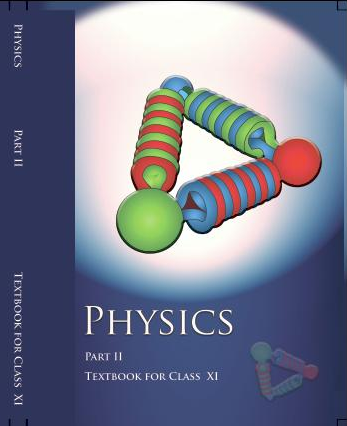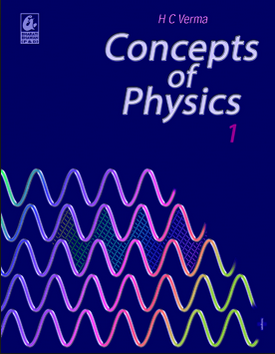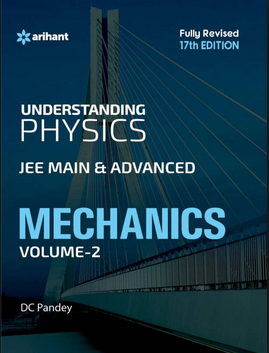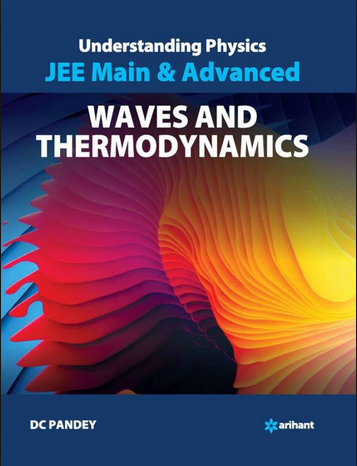# Oscillations and Waves (Weightage 3%)   Share

### Topics from Oscillations and Waves

• Periodic motion: period, frequency, displacement as a function of time (4 concepts)
• Simple harmonic motion (S.H.M.) and its equation; phase (18 concepts)
• energy in S.H.M. kinetic and potential energies (6 concepts)
• oscillations of a spring -restoring force and force constant (5 concepts)
• Simple pendulum derivation of expression for its time period (13 concepts)
• Free, forced and damped oscillations, resonance. (6 concepts)
• Wave motion: Longitudinal and transverse waves (8 concepts)
• the speed of a wave. Displacement relation for a progressive wave. (31 concepts)
• Beats, Doppler effect in sound. (11 concepts)
• The principle of superposition of waves, the reflection of waves, (7 concepts)
• Standing waves in strings and organ pipes, fundamental mode and harmonics (11 concepts)
• Simple harmonic motion ( SHM ) and its equation (1 concepts)
• Oscillation in a spring (3 concepts)
• Sound Wave (13 concepts)
• waves on a string (15 concepts)
• Pendulum and its time period (9 concepts)
• Simple harmonic motion ( SHM ) (8 concepts)
• Damped and forced oscillations (2 concepts)

### Important Books for Oscillations and Waves

••••Exams
Articles
Questions